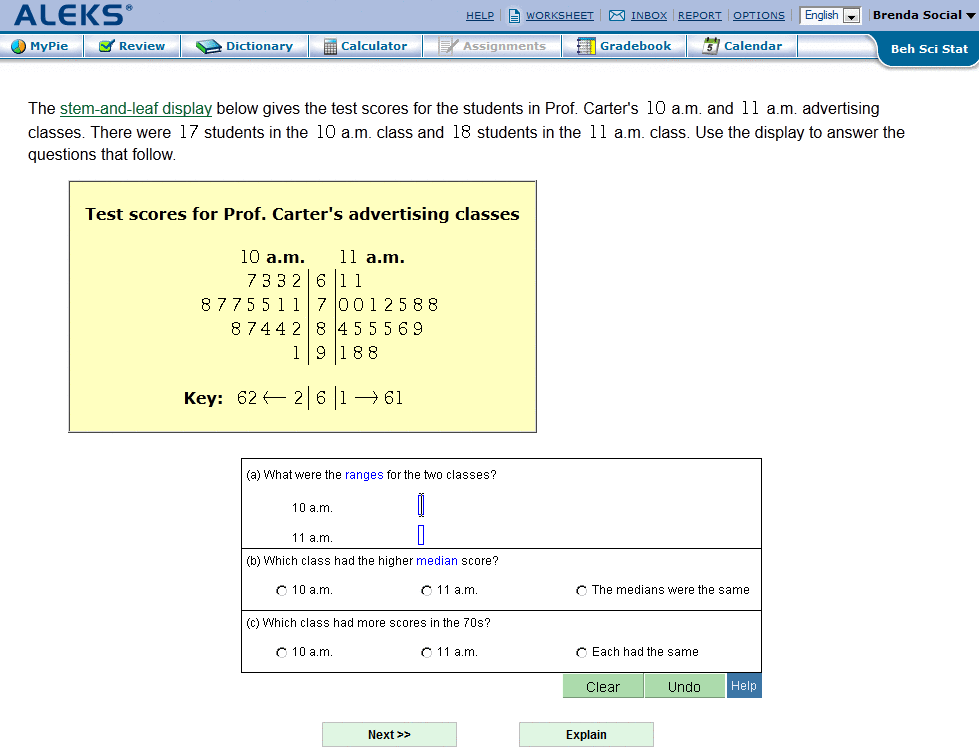5.3.1 Practice PageFigure 5.4: Practice Page
Clicking on the name of a topic from the student's pie chart will display a page containing an instance of the problem, followed by the Answer Editor. This is where a solution to the problem can be attempted (Fig. 5.4). All practice problems are generated by algorithms, with randomly selected numerical values, so that the variety of problem instances for any topic is very high.
Below the Answer Editor are buttons labeled Next and Explain. Clicking on Next has the same effect as described for the Assessment Mode: it submits the answer. Here, however, the user is given immediate feedback on their answer (Sec. 5.4). If correct, the student will receive a congratulatory message. Next, a new problem is presented. In the case where the topic is considered mastered, the student will receive two options; the student can choose to click Done to move on to a different topic, or they can click More Practice to practice the topic further.
When the student enters an incorrect answer, ALEKS will return the presentation of the original problem with feedback on the student's error. Students can then click the Explain button.